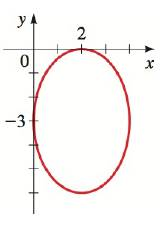Chapter 12.4, Problem 32E### Algebra and Trigonometry (MindTap ...

4th Edition
James Stewart + 2 others
ISBN: 9781305071742

#### Solutions

Chapter
Section### Algebra and Trigonometry (MindTap ...

4th Edition
James Stewart + 2 others
ISBN: 9781305071742
Textbook Problem

# SKILLS 29-34 ■ Finding the Equation of a Shifted Conic Find an equation for the conic whose graph is shown.To determine

To find:

The equation of the conic.

Explanation

Given:

The graph of the conic section is given below.

Figure (1)

Approach:

For an equation in x and y, if the value of x is replaced by x±h, then the graph is shifted horizontally and if the value of y is replaced by y±k, then the graph is shifted vertically.

The equation of an ellipse centred at (h,k) is given by the following formula.

(xh)2a2+(yk)2b2=1(1)

Calculations:

Consider the graph of the conic.

The major axis is the vertical axis.

The coordinates of the major axis are (2,0) and (2,6).

The center of the ellipse is the midpoint of the major axis.

Therefore, the center of the ellipse is given below.

(2+22,062)=(2,3)

The length of the major axis is given below.

0(6)=6

Therefore, the value a is given below.

a=62=3

The coordinates of the minor axis are (0,3) and (4,3)

### Still sussing out bartleby?

Check out a sample textbook solution.

See a sample solution

#### The Solution to Your Study Problems

Bartleby provides explanations to thousands of textbook problems written by our experts, many with advanced degrees!

Get Started